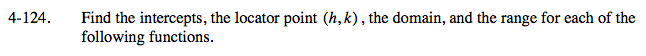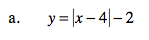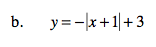### Home > A2C > Chapter 4 > Lesson 4.2.4 > Problem4-124

4-124.General equation: y = a|xh| + k

To find the x-intercept(s), let y = 0.
To find the y-intercept(s), let x = 0.

How does the negative sign affect the graph?Use the eTool below to graph the equations to answer the problem.
Click the link at right for the full version of the eTool: A2C 4-124 HW eTool